# 15-344/Homework Assignment 10

This assignment is due by Friday December 11 at 5PM. It can be submitted either to Gaurav during his office hours on Thursday December 10 at 3:30-5:30 or during his office hours on Friday December 11 at 4-5, both at 215 Huron room 1012. It can also be submitted to a drop box near Dror's office, Bahen 6178, at any time between Thursday December 10 at 5 and Friday December 11 at 5.

Here and everywhere, neatness counts!! You may be brilliant and you may mean just the right things, but if the teaching assistants will be having hard time deciphering your work they will give up and assume it is wrong.

Solve and submit your solutions of the following three problems:

Problem 1. What is the probability that a soccer game with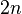$2n$ goals ends with a tie, and that this tie is the first tie in the game (except before the first goal)?

Problem 2. How many possible histories are there for a soccer game that ends with the score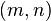$(m,n)$, where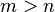$m>n$, if it is known that the first team is never behind the second?

Problem 3. Let$n$ be a natural number. How many sequences of integers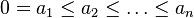$0=a_1\leq a_2\leq\ldots\leq a_n$ are there, such that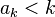$a_k for every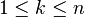$1\leq k\leq n$? For example, for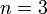$n=3$ the allowed sequences are 000, 001, 002, 011, and 012.

 Dror's notes above / Students' notes below

Homework Assignment 10 Solutions

1)What is the probability that a soccer game with$2n$ goals ends with a tie, and that the tie is the first tie in the game (except before the first goal)?

A)$2n$ goals ending with a tie means$n$ goals each (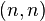$(n,n)$ on the Catalan diagram). Number of possibilities with no tie before$(n,n)$ is basically the number of possible paths to$(n,n)$ without crossing the diagonal line. This is equal to: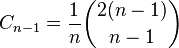$C_{n-1} = \frac{1}{n}\binom{2(n-1)}{n-1}$

Total number of possible games with$2n$ goals is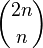$\binom{2n}{n}$

The required probability is: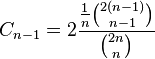$C_{n-1} = 2\frac{\frac{1}{n}\binom{2(n-1)}{n-1}}{\binom{2n}{n}}$

The reason why we multiply the probability by two is because there are two possible cases (either above the diagonal or below the diagonal).

2)How many possible histories are there for a soccer game that ends with the score$(m,n)$, where$m>n$, if it is known that the first team is never behind?

A) This can be thought of as an A-dominant game. The total number of possibilities with end score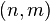$(n,m)$ is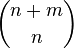$\binom{n+m}{n}$ Using Andre's Reflection, we need to find the number of good paths (A-dominant paths): Total paths - Bad paths (any paths that cross the diagonal) = Good Paths.

Number of Bad Paths to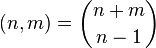$(n,m) = \binom{n+m}{n-1}$, so using Andre's Reflection, we have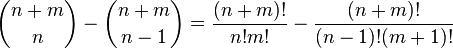$\binom{n+m}{n} - \binom{n+m}{n-1} = \frac{(n+m)!}{n!m!} - \frac{(n+m)!}{(n-1)!(m+1)!}$

Simplifying it gives: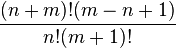$\frac{(n+m)!(m-n+1)}{n!(m+1)!}$

3) Let$n$ be a natural number. How many sequences of integers$0=a_1\leq a_2\leq\ldots\leq a_n$ are there, such that$a_k for every$1\leq k\leq n$? For example, for$n=3$ the allowed sequences are 000, 001, 002, 011, and 012.

A) This could also be thought as an A-dominant game. We can think of each digit in a sequence as the score difference between the two teams at a certain point in the game. For example,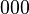$000$ would mean the score started with a tie and ended with a tie. Likewise,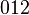$012$ would mean that (starting from the right end), Team A led by two goals in the first third of the game, by one goal at half time and gave up another goal and tied the game in the end. In the 3 digit sequence case, the total goal in the game is 3 therefore sequences like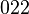$022$ are not possible. (this would mean Team B was down by two goals and then scored two to tie the game -> 4 goals total). Therefore, for sequences of length$n$, the number number of sequences is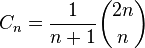$C_{n} = \frac{1}{n+1}\binom{2n}{n}$